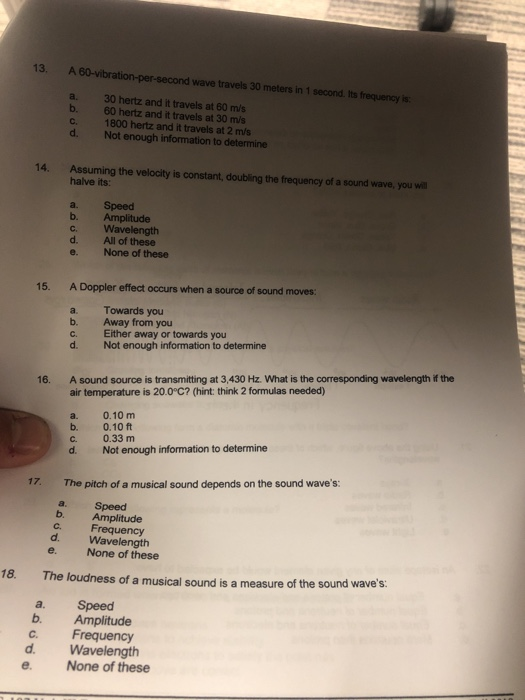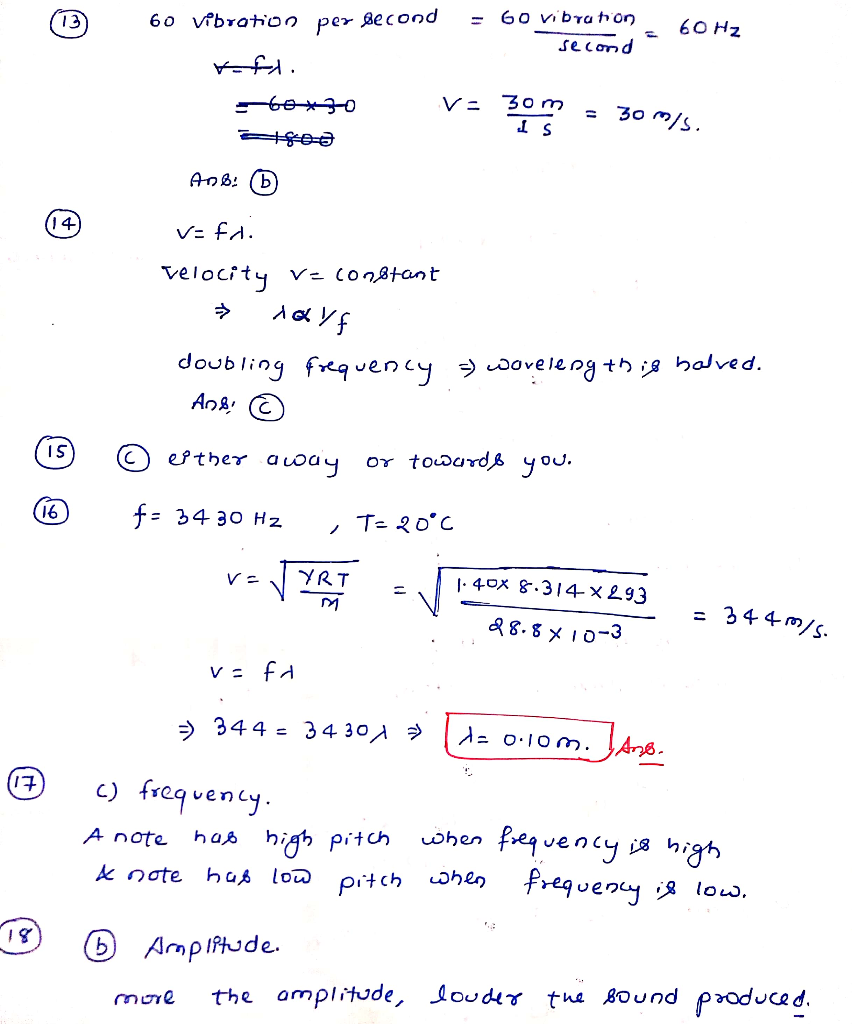# A 60-vibration-per-second wave travels 30 meters in 1 second. its frequency is13. A 60-vibration-per-second wave travels 30 meters in 1 second. Its frequency is 30 hertz and it travels at 60 m/s 60 hertz and it travels at 30 m/s 1800 hertz and it travels at 2 m/s Not enough information to determine Assuming the velocity is constant, doubling the frequency of a sound wave, you will halve its: b. C d. Speed Amplitude Wavelength All of these None of these 15. A Doppler effect occurs when a source of sound moves: Towards you Away from you C. Either away or towards you d. Not enough information to determine b. 16. A sound source is transmitting at 3,430 Hz. What is the corresponding wavelength of the air temperature is 20.0°C? (hint: think 2 formulas needed) a. b. c. d. 0.10 m 0.10 ft 0.33 m Not enough information to determine The pitch of a musical sound depends on the sound wave’s: b. C. Speed Amplitude Frequency Wavelength None of these e. 18. The loudness of a musical sound is a measure of the sound wave’s: a. b. Speed Amplitude Frequency Wavelength None of these

Also Read :   What type of hard drive has no moving parts making it fast and durable

vibru tion = 60 60 Hz second 60 vibration 13 per second = 30 m/s. AnB: (b) 14) V: fd. velocity v= con8tant doubling frequency > woveleng th is balved. And: or towardß you. I5 esther auday (16 f= 34 30 Hz T= 20°C YRT J. 40x 8.314×293 = 344 m/s. 88.8×10-3 > 344 = 34 30A > d= 0:10m. Aso. c) frequency. 17) when frequency is high frequery is low. has high Pitch A note & note has low pitch when 18) 6 AmpIftude. the amplitude, louder twe Bound produced. more